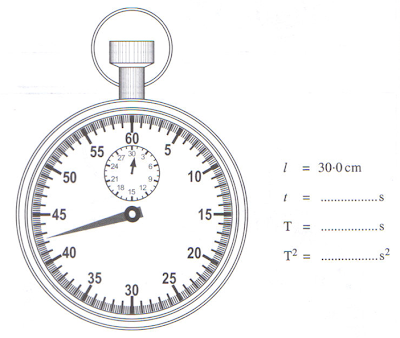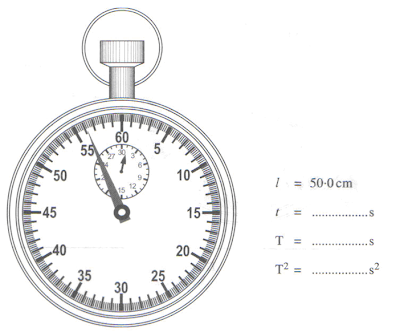Saturday, June 6, 2009

SPM 2008 Paper 3 No. 1

A students carries out an experiment to investigate the relationship between the length, l , of a simple pendulum and the period of oscillation, T. The apparatus set-up for this experiment is shown in Diagram 1.The student starts the experiment with a pendulum length of 30.0 cm. The pendulum bob is displaced and then released so that it oscillates. The time taken, t, for 10 complete oscillations is recorded. The experiment is repeated with pendulum lengths of 40.0 cm, 50.0 cm, 60.0 cm and 70.0 cm.
The corresponding stopwatch readings for 10 complete oscillations are shown in Diagram 5.2, 5.3, 5.4 , 5.5 and 5.6.(a) For the experiment described on pages6, 7and 8, identify:

(i) The manipulated variable

………………………………………………………………………………………..[1 mark]

(ii) The responding variable

……………………………………………………………………………………...[1 mark]

(iii) The constant variable

……………………………………………………………………………………...[1 mark]

(b) For this part of question, write your answers in the spaces provided in the corresponding Diagrams. Your answers must be correct to two decimal places.
Based on Diagrams 5.2, 5.3, 5.4, 5.5 and 5.6:
(i) Record the readings, t, of the stopwatch. [2 marks]

(ii) For each value of t in 5(b)(i), calculate the period of oscillation, T, of the pendulum using the following equation:
T = t/10
Record the value of T. [1 mark]

(iii) Calculate T2 for each value of T in 5(b)(ii). Record the value of T2. [2 marks]

(c) Tabulate your results for all values of l, T and T2 in the space below.

[2 marks]

(d) On the graph paper on page10, plot a graph of T2 against l. [5 marks]

(e) Based on your graph in 5(d), state the relationship between T2 and l.

……………………………………………………………………………………….[1 mark]

Responding variable: Time for 10 complete oscillation/ t / Period / T
Constant variable : mass of bob // gravitational acceleration1. Show T2 on the vertical –axis (y-axis) and l on the horizontal – axis (x-axis)
2. State the units of the variables correctly
3. Both axes are marked with uniform scale
4. All five points are plotted correctly
5. Best fit line is drawn
6. Show the minimum size of graph at least 5 x 4 (2 cm x 2 cm) squares

T2 is directly proportional to l